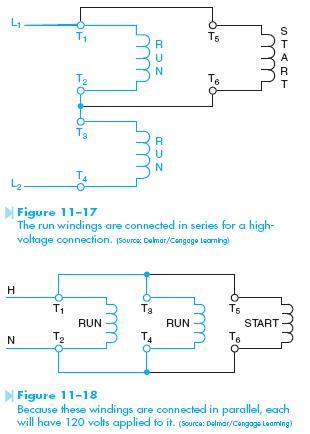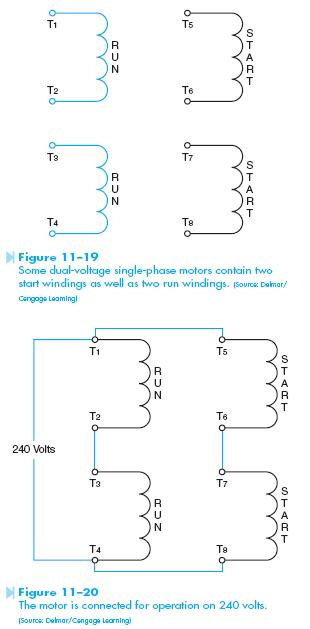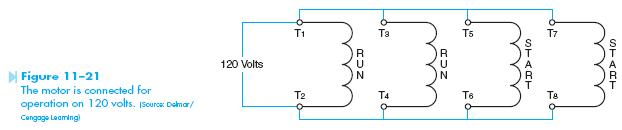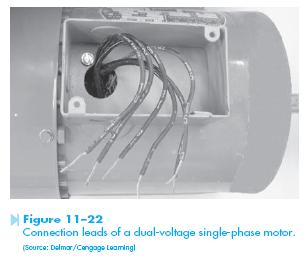# Dual Voltage Motor

Single-phase motors can also be constructed to operate on two separate voltages. These motors are designed to be connected to 120 or 240 volts. A common connection for this type of motor contains two run windings and one start winding, Figure 11–17. The run windings are labeled T1–T2, and T3–T4. The start winding is labeled T5 and T6. In the circuit shown in Figure 11–17, the windings have been connected for operation on a 240-volt line. Each winding is rated at 120 volts. The two run windings are connected in series, which causes each to have a voltage drop of 120 volts when connected to 240 volts. Notice that the start winding has been connected in parallel with one of the run windings. This causes the start winding to have an applied voltage of 120 volts also. Notice that each of the windings has 120 volts connected to it, which is the rating of the windings.If the motor is to be operated on a 120 volt line, the windings are connected in parallel as shown in Figure 11–18. Because these windings are connected in parallel, each will have 120 volts applied to it.

Some dual-voltage motors will contain two start windings as well as two run windings, as shown in Figure 11–19. The run windings are labeled T1 through T4, the same as dual-voltage motors that contain only one start winding. One of the start windings is labeled T5 and T6. The second start winding is labeled T7 and T8. If the motor is to be connected for operation on 240 volts, the run windings are connected in series by connecting T2 and T3 together, and the start windings are connected in series by connecting T6 and T7 together. The start windings are then connected in parallel with the run windings, as shown in Figure 11–20. The direction of rotation can be changed by reversing T5 and T8.If the motor is operated on 120 volts, the run and start windings are connected in parallel as shown in Figure 11–21. If the motor is to be reversed, leads T5 and T7 are changed with leads T6 and T8. Some manufacturers label the start winding leads T5 and T8 even if they contain only one start winding. The connection leads of a dual-voltage single-phase motor are shown in Figure 11–22.## One Reply to “Dual Voltage Motor”

1.Lisias Ngwena says:

how can i connect a dual voltage motor on cam swtich